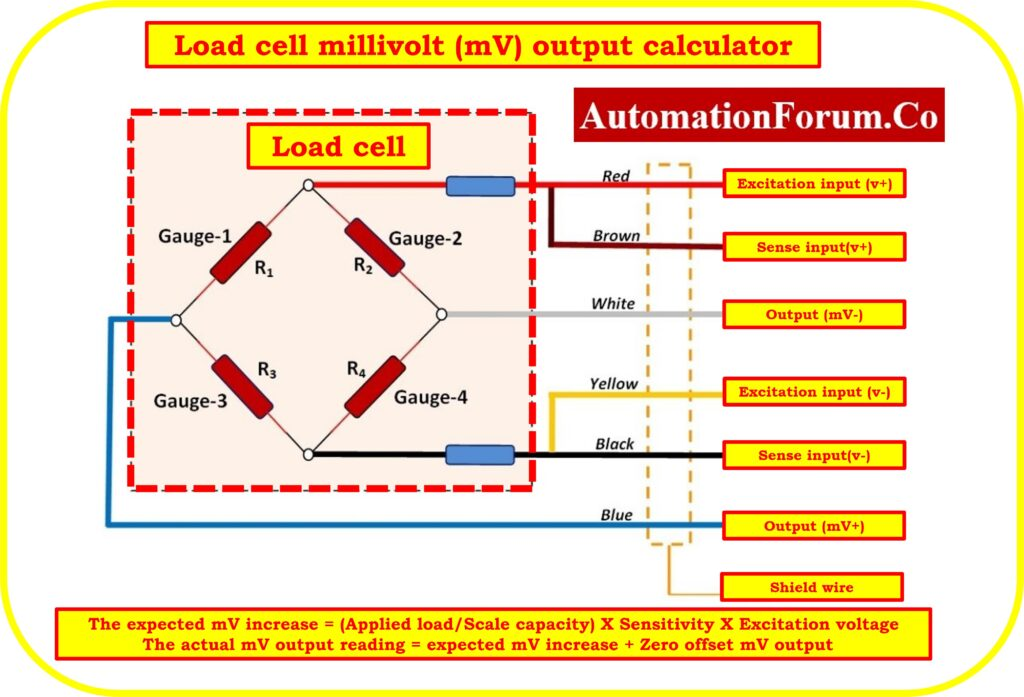# Load cell millivolt (mV) output calculator## How can I calculate the expected millivolt reading of my scale?

To determine a load cell’s millivolt (mV) output, However, the following details are still necessary.  Such are

## Sensitivity of the Load Cell (mV/V):

The sensitivity of a load cell refers to the change in millivolts produced by the load cell per unit change in applied load. It is usually provided by the load cell manufacturer and is expressed in millivolts per volt (mV/V). The sensitivity value helps us determine the relationship between the applied load and the millivolt output.

## Excitation Voltage (V):

The excitation voltage is the voltage supplied to the load cell for proper operation. It is typically specified by the load cell manufacturer and is given in volts (V). The excitation voltage is necessary for converting the mechanical force exerted on the load cell into an electrical signal.

The applied load refers to the weight or force you place on the scale for measurement. It can be in various units such as kilograms, pounds, or Newtons, depending on the load cell’s specifications. It’s important to ensure that the units of the applied load match the sensitivity of the load cell.

## Scale capacity(Kg):

The full capacity of a weigh scale refers to the maximum weight that the scale is capable of measuring accurately and reliably. It represents the upper limit of the scale’s weight range, beyond which it may provide inaccurate readings or risk damaging the equipment.

## Zero offset mv output (mV):

Zero offset mV output from a scale refers to the electrical signal produced by the scale’s load cells when there is no weight applied. It represents the baseline voltage level, allowing for calibration and accurate measurement of weight by comparing the difference between the zero offset and actual output.

## Formula for the calculation

Use the following formula in order to determine the millivolt reading that should be expected:

## Example calculation

The expected mV increase = (Applied load/Scale capacity) X Sensitivity X Excitation voltage

The actual mV output reading = expected mV increase + Zero offset mV output

## Step 1: Determine the Sensitivity:

Consult the load cell’s datasheet or manual to find the sensitivity value. For example, let’s assume the sensitivity is specified as 2 mV/V.

## Step 3: Measure the Applied Load:

Place the test weight on the scale and measure the applied load using a calibrated instrument. Ensure that the units of the applied load match the sensitivity of the load cell. For instance, let’s assume you place a 1000 kg test weight on the scale.

## Step 4: Find the Scale capacity:

Consult the load cell’s datasheet or manual to find the scale capacity. For example, let’s assume the scale capacity is 5000 Kg.

## Step 5: Zero offset mv output:

The weighing control display unit may show the zero offset mV output signal from a scale. Additionally, it can be physically measured using a multimeter from the average card output of a load cell or from the load cell input signal terminal in the weighing control display unit. For example, let’s assume the 1.5mV

The expected mV increase= (Applied load/Scale capacity)X Sensitivity X Excitation voltage

= (1000/5000) X 2.00 X 5.00

The expected mV increase = 2.00 mV

The actual mV output reading = expected mV increase +Zero offset mV output

The actual mV reading = 1.5 + 2.00

The actual mV reading= 3.50 mV

So the load cell output from the weighing scale will be 3.50 mV

## Load cell millivolt (mV) output calculator

The following calculator was used to determine the Load cell millivolt (mV) output when placing the test weight on the weighing platform during weighing system troubleshooting.